## Sunday, March 28, 2021

### New variable rate ML method for quantitative traits in phytools

For part of a project I'm involved in, I just added a new function to the phytools package, `multirateBM`, that uses likelihood to fit a multi-rate Brownian motion model in which the (logarithm of the) rate evolves by a Brownian process, and every edge of the tree can consequently have a slightly different value of σ2.

The code of the function can be viewed here, and you can use `multirateBM` just by updating phytools from it's GitHub page with the package devtools as follows:

``````devtools::install_github("liamrevell/phytools")
``````

To show how the function works, I'll first simulate some data under a variable-rate model. I'll fit my model, and then I'll compare the estimated vs. true rates.

First, here are some data:

``````library(phytools)
tree<-pbtree(n=26,tip.label=LETTERS)
sig2<-exp(fastBM(tree,internal=TRUE))
tt<-tree
tt\$edge.length<-tt\$edge.length*apply(tt\$edge,1,
function(e,x) mean(x[e]),x=sig2)
x<-fastBM(tt)
``````

Here's a plot of the tree in which the edges have been stretched to be equal to time × the evolutionary rate on each edge:

``````plotTree(tt)
``````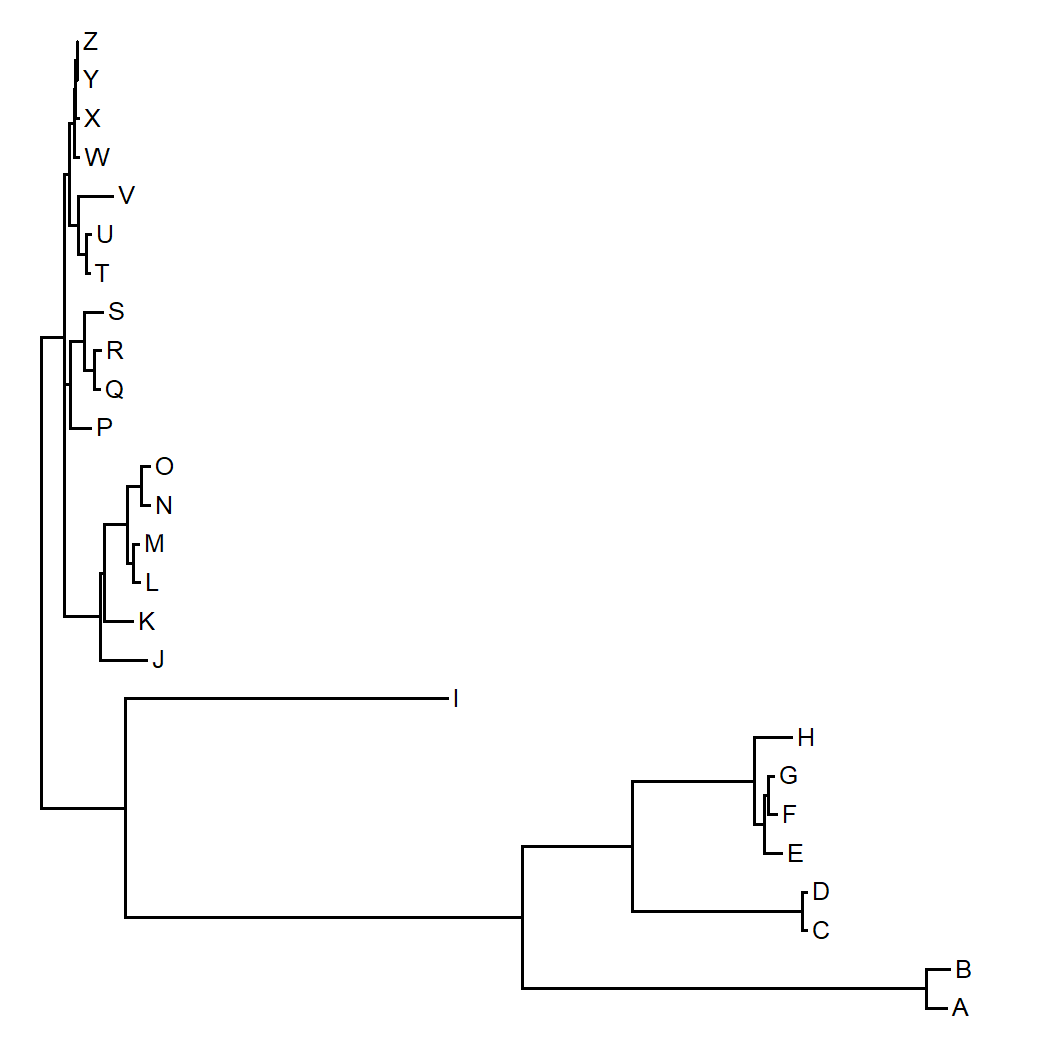We can also see this pretty easily using a `contMap`-style visualization:

``````contMap(tree,x)
``````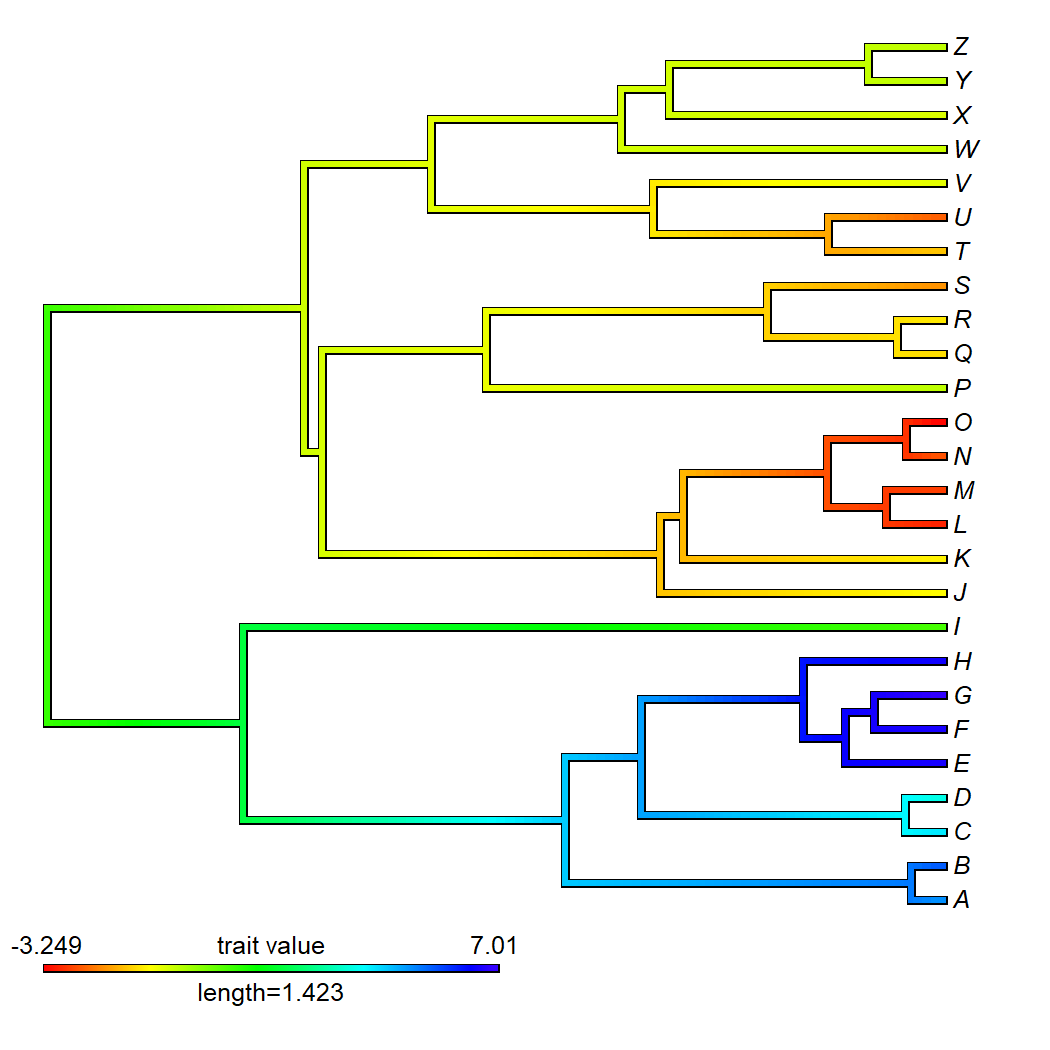OK, now let's fit our model.

I'll do that using `multirateBM` as follows:

``````fit.ml<-multirateBM(tree,x,n.iter=3)
``````
``````## Beginning optimization....
## Optimization iteration 1.
## Optimization iteration 2.
## Optimization iteration 3.
## Done optimization.
``````
``````fit.ml
``````
``````## Multi-rate Brownian model using multirateBM.
##
## Fitted rates:
##         A        B       C        D       E        F        G        H
##  1.862594 1.871276 1.65685 1.671728 1.08921 1.053945 1.068177 1.211217 ....
##
## log-likelihood:  -54.439295
## AIC:  212.878589
##
## R thinks it has found a solution.
``````

I built a handy `plot` method to visualize our results. Let's use it:

``````plot(fit.ml,digits=3)
``````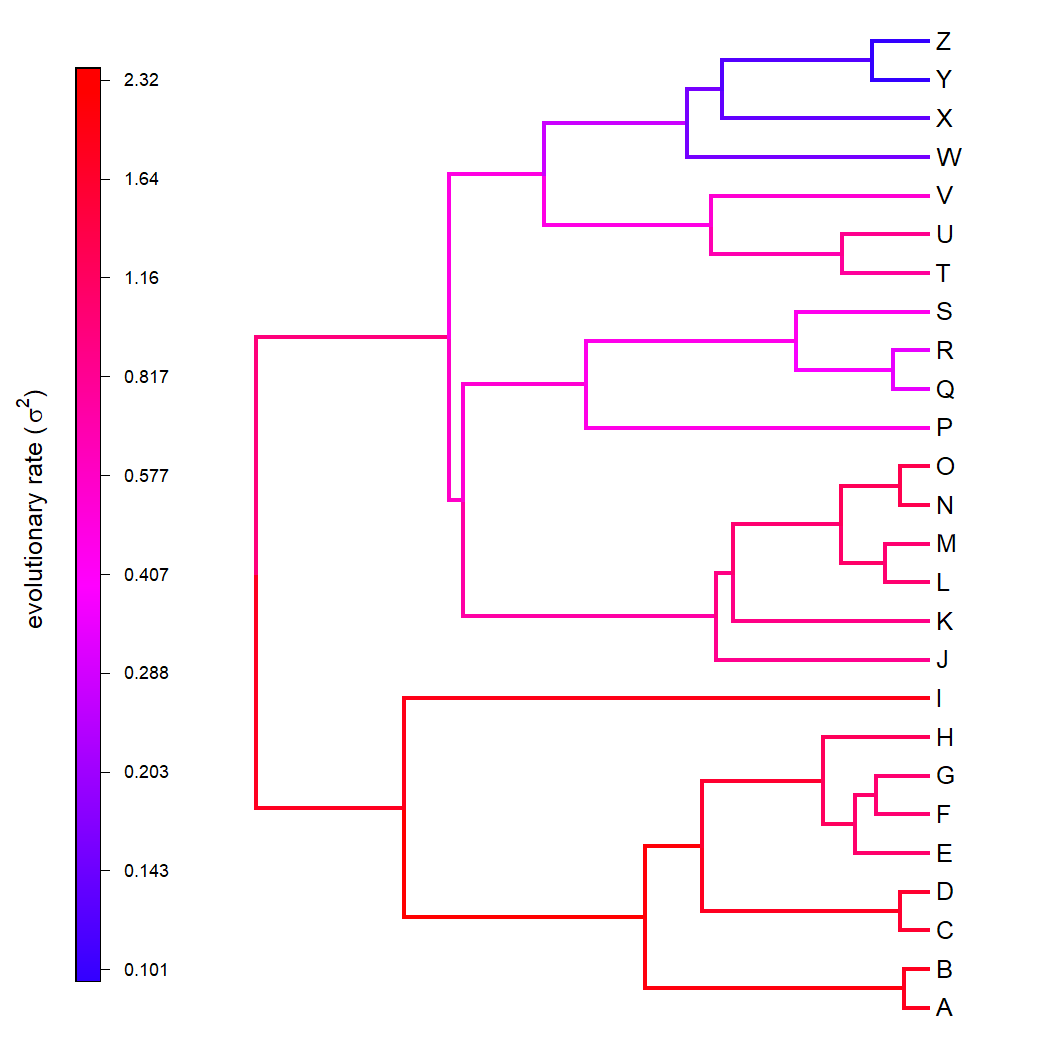Finally, we can compare our simulated & estimated σ2 values as follows:

``````## generating sigma^2 by edge
SIG2<-apply(tree\$edge,1,function(e,x)
mean(x[e]),x=sig2)
## estimated sigma^2 by edge
est.SIG2<-apply(tree\$edge,1,function(e,x)
mean(x[e]),x=fit.ml\$sig2)
xylim<-range(c(SIG2,est.SIG2))
par(mar=c(5.1,5.1,4.1,1.1))
plot(SIG2,est.SIG2,pch=21,bg="grey",cex=1.5,bty="n",
xlab=expression(paste("true rates, ",sigma^2)),
ylab=expression(paste("estimates rates, ",sigma^2)),
log="xy",xlim=xylim,ylim=xylim)
lines(xylim,xylim,lwd=2,
col=make.transparent(palette(),0.5))
title(main="Simulated vs. Estimated Brownian Rates",
font.main=3)
``````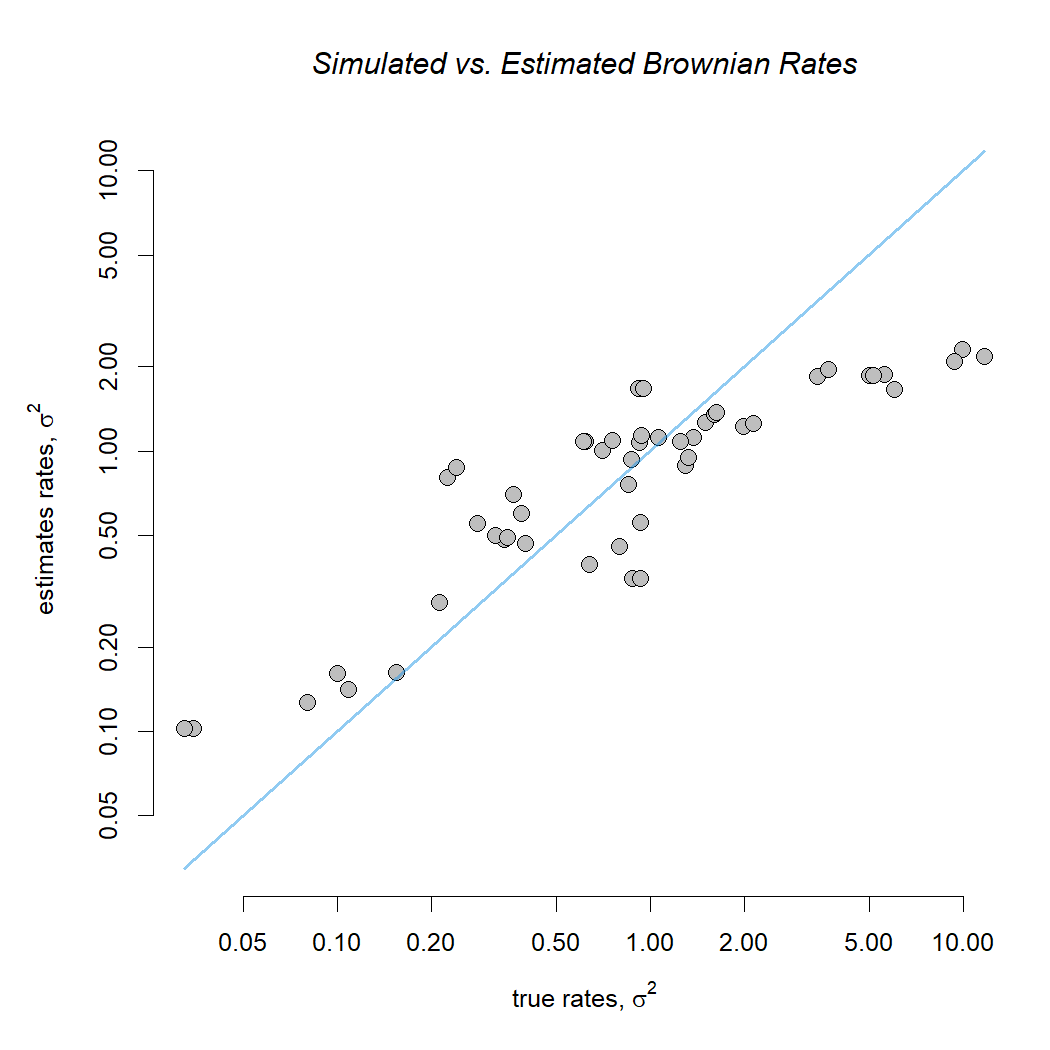Honestly, I was shocked (shocked!) at how well this worked with simulated data.

To see that it also works (or, at least, runs) with real data, let's load some & try it.

These are a phylogeny and body mass data for mammals.

``````data(mammal.tree)
data(mammal.data)
lnMass<-setNames(log(mammal.data\$bodyMass),
rownames(mammal.data))
fit.mammal<-multirateBM(mammal.tree,lnMass,n.iter=3)
``````
``````## Beginning optimization....
## Optimization iteration 1.
## Optimization iteration 2.
## Optimization iteration 3.
## Done optimization.
``````
``````fit.mammal
``````
``````## Multi-rate Brownian model using multirateBM.
##
## Fitted rates:
##  U._maritimus U._arctos U._americanus N._narica P._lotor M._mephitis M._meles C._lupus
##       0.01004  0.009619      0.139263   0.02582 0.016295    0.136526 0.010344 0.190132 ....
##
## log-likelihood:  -231.849096
## AIC:  659.698193
##
## R thinks it has found a solution.
``````

We'll plot it with a black background just for fun.

``````par(bg="black",fg="white")
plot(fit.mammal,fsize=0.8,digit=2,ftype="i")
``````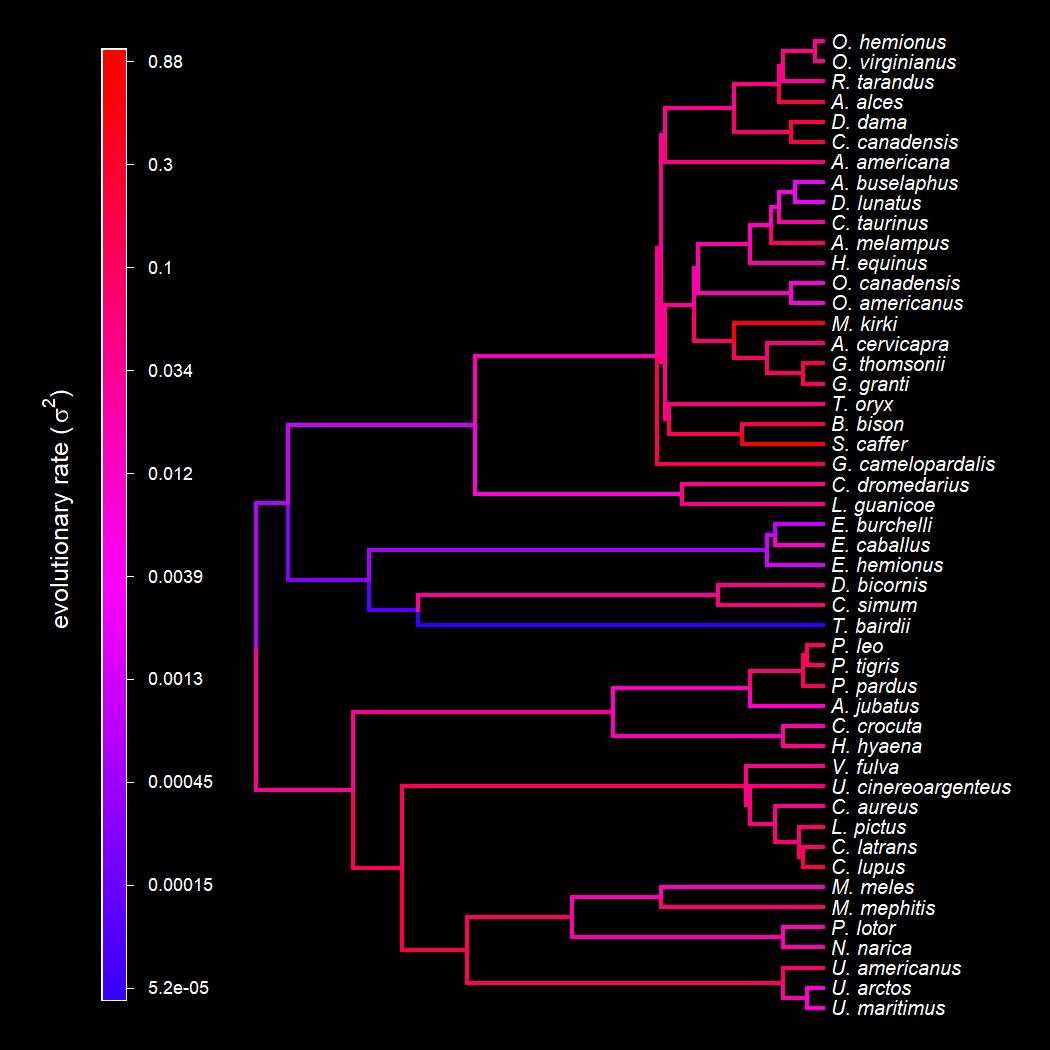Cool.

#### 1 comment:

1.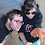This comment has been removed by the author.

Note: due to the very large amount of spam, all comments are now automatically submitted for moderation.